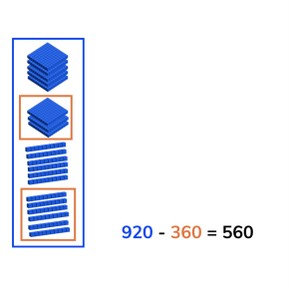Subtraction to 1,000 crossing tens

# Subtraction to 1,000 crossing tens

Subtract numbers to 1,000 crossing tens.8,000 schools use Gynzy92,000 teachers use Gynzy1,600,000 students use Gynzy

## General

Learn how to subtract numbers to 1,000 crossing tens.

## Standards

CCSS.Math.Content.2.NBT.B.7

## Learning objective

Students will be able to subtract numbers to 1,000 crossing tens.

## Introduction

Ask students to solve the problems on the interactive whiteboard. Ask them to explain their strategy. To check their answers, have students "saw" the piece of fruit. Drag the top image of the fruit away to show the answer on the halved fruit.

## Instruction

Explain that you can subtract by counting. You first count how many there are in total, this is the first number of the subtraction problem (the minuend). Then you count how many are taken away, which is the second number of the subtraction problem (the subtrahend). When you take the second from the first you are left with the difference. Show this on the interactive whiteboard using the blocks, and discuss the different problems. This includes problems in which the second number (the subtrahend) has hundreds, tens, and ones. Check that students are able to solve a few problems on their own or in pairs with the given problems with blocks.Ask them to say the problem they are solving out loud, and to say the steps they are taking out loud. Next explain that you can solve these subtraction problems in different ways. Show that you can use a number line to subtract. You can also split the subtrahend and take away the hundreds, tens, and ones in steps. You can also choose to take away the subtrahend in one step. Emphasize to students that they should first carefully look at the problem and then decide which method to use, because all of them work, but some are easier to use on different math problems. Ask students to solve a few problems individually or in pairs, explaining their strategy. Finally walk through the steps of a story problem with the class. Explain that you first determine what kind of math problem it is, then you determine which numbers are important for the problem. You say or write the problem and finally solve the problem. Do one story problem as a class and then ask students to solve another individually or in pairs.

Check that students are able to subtract numbers to 1,000 crossing tens by asking the following questions:
- How do you solve story problem?
- How do you solve 380 - 110?

## Quiz

Students are given problems in which they must solve using visual support, solve an abstract problem, and story problems. Encourage students to explain their strategy and walk through the steps of the subtraction problems.

## Closing

Discuss with students that it is important to subtract to 1,000 crossing tens so you can determine how much you have left. Ask students to solve the problems on the interactive whiteboard. Ask students to explain their strategy. Which method do they use?
Finally ask students to solve the final three problems on the interactive whiteboard. Repeat what you did at the start of the lesson and "saw" the fruit.

## Teaching tips

Students who have difficulty with subtraction can make use of the number line to support their subtraction. Remind students that they can take different sized steps on the number line.

## Instruction materials

MAB blocks or manipulatives, number line

### The online teaching platform for interactive whiteboards and displays in schools

• Save time building lessons

• Manage the classroom more efficiently

• Increase student engagement